## Hierarchical Clustering in Python

##### In this part of Learning Python we Cover Hierarchical Clustering Technique In Python
Written by |08-May-2019 | 0 Comments | 797 Views

Hierarchical Clustering is another form unsupervised form learning. It is also called hierarchical cluster analysis. It is an algorithm that groups similar objects into groups called clusters. The endpoint is set of a cluster, where each cluster is distinct from each other cluster, the object within a cluster are similar to one another and have the minimum distance between them.

Hierarchical Clustering builds a tree-based hierarchical taxonomy that is known as dendrogram from the set of feature vector x.Figure 1

A dendrogram is a tree that shows how clusters are merged/split hierarchically. In the dendrogram, each node on the tree is a cluster, and each leaf node is a singleton cluster.

Approaches:

There are two approaches to solve the hierarchical clustering:

• Agglomerative Clustering Algorithm
• Divisive Clustering Algorithm

Agglomerative Clustering Algorithm:

This is the bottom-up approach of a hierarchical clustering algorithm. Initially, all the data of feature vector x is a single cluster. Then it merges the two closest cluster by computing the distance matrix and repeat this procedure until only single clusters remain.

Divisive Clustering Algorithm:

It is the second approach and opposite to the first one, but the result of making a tree of a cluster is the same. It is a top-down approach. Initially, all the data is a single cluster and recursively split the data until only one feature as cluster remains.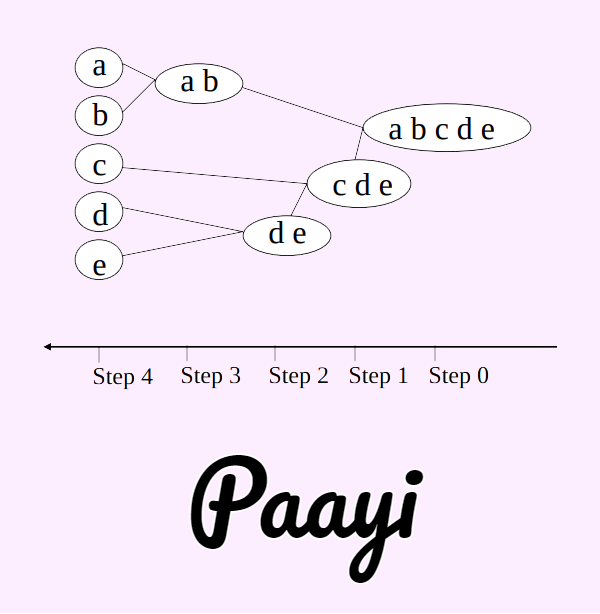Figure 2

How Does It Work?

As mentioned above that each cluster is merged based on the distance matrix. However, there are many variations of finding the closest pair of clusters.

1. Closest Points between Clusters: The distance between two clusters is defined as the smallest distance between a pair of the data points within the clusters. This is also known as the single linkage.

Using the maximum similarity pair by the formula we get:

since,c=maxx∈ci,y∈cjsimx,y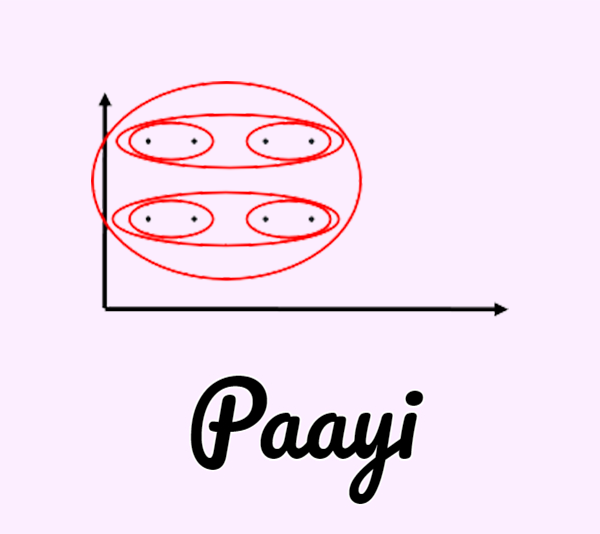Figure 3

Single linkage cluster form thin and sleek clusters due to chaining effect.

1. Farthest Point between Clusters: The distance between two clusters is defined as the most considerable distance between a pair of the data points within the clusters. This is also known as the complete linkage.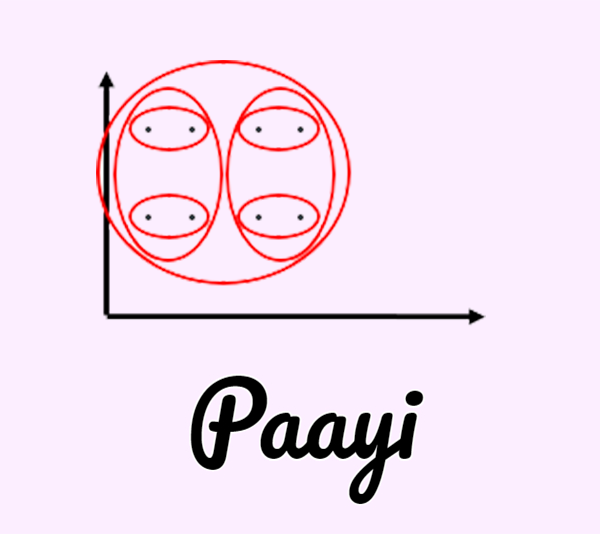Figure 4
2. Using minimum similarity pairs by the formula :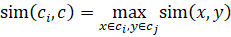It makes the tighter and spherical clusters which are preferable.

Average Pairwise distance Between Clusters: As its names indicate. The distance between two clusters is defined by the average distance between a pair of data points within the clusters.

1. Ward’s Method: Similarity of two clusters is based on the increase in squared error when two clusters are merged. It is similar to the group average if the distance between points is distance squared.

When to use When:

• A single link can handle non-elliptical data but sensitive to noisy data.
• The full link is less susceptible to noise and outliers but is biased toward globular structure and tends to break large clusters.
• Group average is generalized for both single and complete. It is less susceptible to noise and outliers and biased toward globular clusters.

Example:

Suppose we have a data set given as below:

x = {(0,0), (1,2), (2,1), (4,1), (5,0), (5,3)}

if we plot the data it looks like this:Figure 5

1st  we will make the distance matrix of all the given feature and will take with the minimum.

Suppose the distance of (1,2) and (2,1) will be (1.5, 1.5) and update the distance matrix.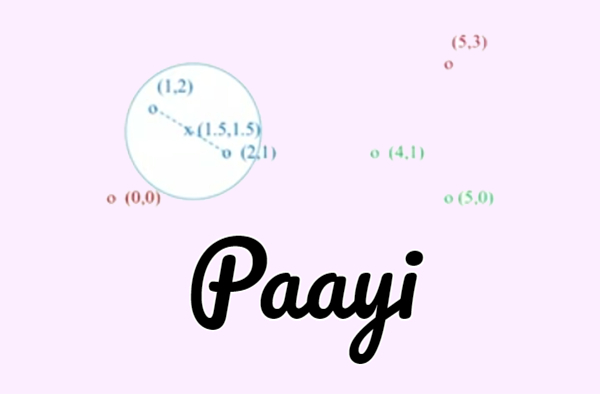Figure 6

Respectively we will find minimum value from distance matrix again this time take (4,1) and (5,0)

The distance of both the points will be (4.5, 0.5) and a new cluster will be formed: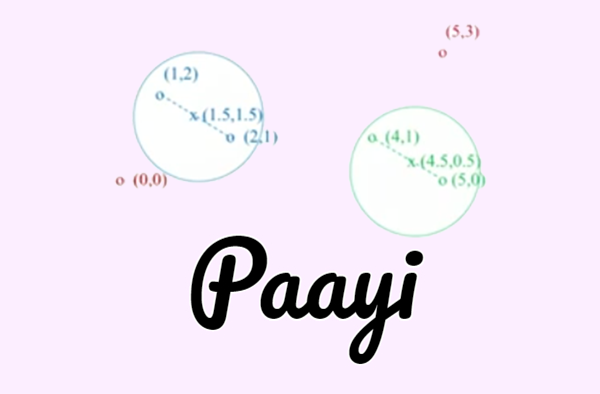Figure 7

Again this procedure now we will take an average of (1,2),(2,1) and (0,0) the output will be (1, 1)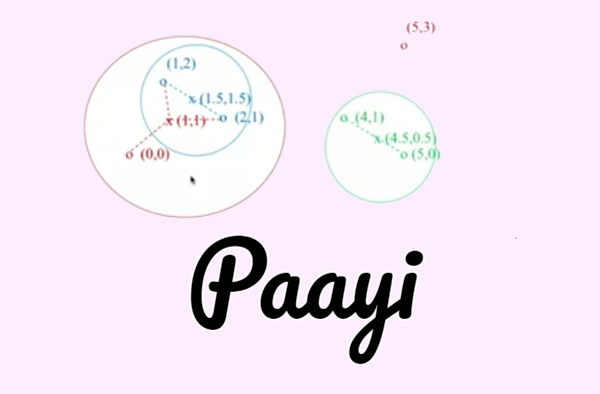Figure 8

Than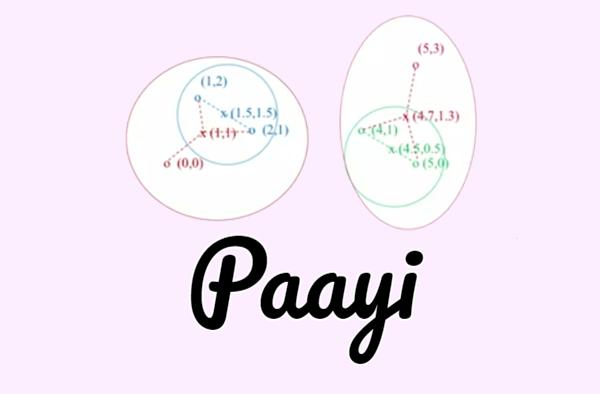Figure 9

At the end it will form one cluster and dendrogram will look like the following tree: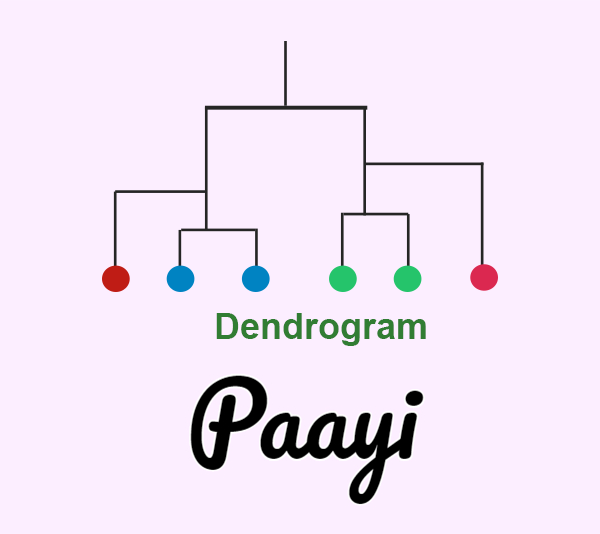Figure 10

Use Cases:

• Crime Location Identification
• Document Classification
• Search Optimization
• Call Record Data analysis.

• Do not have to assume the number of clusters. Any desired number of a cluster can be obtained by cutting the dendrogram at a proper level.
• It corresponds to better and meaningful taxonomies, which provide a better search function.

• High in time complexity
• No objective function
• Different schemes have different problems
- sensitive to noise
-biased toward globular cluster
-breaking large clusters.
• Once the decision is made to form two cluster, it cannot be undone. ​​​​​​

Improvement:

It can be improved by using it with other algorithms named below:

• CURE (Clustering using Representative)
• BIRCH (Balanced Iterative Reducing and Clustering using Hierarchies )
• ROCK (Robust Clustering using Links)
• CHAMELEON Algorithm
• Bisecting K-Means

Conclusion:

Hierarchical Clustering is a method which seeks to build a hierarchy of clusters. It is majorly used in clustering like Google news, Amazon Search, etc. It is giving a high accuracy but with much more time complexity. It is a tradeoff between good accuracy to time complexity. The quality of a simple hierarchical clustering method suffers from its inability to perform an adjustment, once merge it cannot be split again. However, one thing is that it can be improved by using the algorithms mentioned above.

Practical Implementation of Hierarchical Clustering:

`import matplotlib.pyplot as plt  `
`import numpy as np  `
`import scipy.cluster.hierarchy as shc`
`from sklearn.cluster import AgglomerativeClustering`

First of all import all the modules. In this, we have imported Matplotlib to plot the data to know what clusters we are going to make. The NumPy is imported to convert the data into a NumPy array before feeding the data to the machine learning algorithm. Then we have imported hierarchy, and this module is actually to plot the dendrograms. These dendrograms will help us to know how many clusters we should make. We have already seen how dendrogram looks, but now we will use it on real data.

This module will make the model fit to hierarchical clustering.

`import matplotlib.pyplot as plt  `
`import pandas as pd  `
`import numpy as np  `
`import scipy.cluster.hierarchy as shc`
`from sklearn.cluster import AgglomerativeClustering`

And now we will initialize random data.

`X = np.array([[5,3],  `
`    [9,14],`
`    [14,11],`
`    [23,9],`
`    [29,29],`
`    [84,69],`
`    [70,79],`
`    [59,77],`
`    [69,54],`
`    [79,90],])`

The array is initialized as a NumPy array. We can see the data by plotting.

`plt.scatter(X[:,0],X[:,1])`
`plt.show()`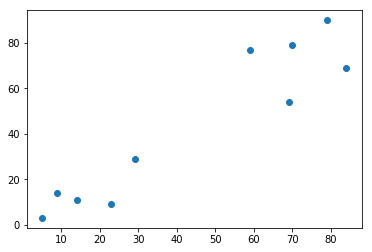Figure 11

The data is too much simple. We can see how many clusters can be made as the data is too much apart. But when the data is complex, we can't predict the number of clusters so that we use the technique of dendrograms. The following is the method:

plt.figure(figsize=(10, 7))
plt.title("Dendrograms")

The dendrogram looks like this:Figure 12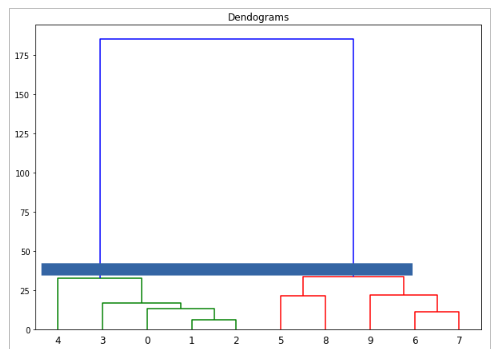Figure 13

In Figure 13, we can see that the blue line is the most continuous line in all the clusters. From here we can deduce that two clusters are the actual clusters.

Now to fit the model following is the method.

`model = AgglomerativeClustering(n_clusters=2, affinity='euclidean', linkage='ward')  `
`model.fit_predict(X) `

The fir and predict first fit the model than predict the classes which in this case is as follows:

 array([1, 1, 1, 1, 1, 0, 0, 0, 0, 0])